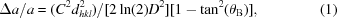## letters to the editorJOURNAL OFAPPLIEDCRYSTALLOGRAPHY
ISSN: 1600-5767
Volume 52| Part 3| June 2019| Pages 693-694
RESPONSE

## Comment on the article The nanodiffraction problem

aInstitute of Physical Chemistry, PAS, Kasprzaka 44/52, 01-224, Warszawa, Poland
*Correspondence e-mail: zkaszkur@ichf.edu.pl

(Received 13 August 2018; accepted 8 May 2019; online 28 May 2019)

The article The nanodiffraction problem (Xiong et al., 2018) discusses the origin of the peak shifts for nanocrystalline fragments of a perfect periodic lattice from their positions determined by Bragg's law. We submit that the article fails, however, in correct analysis of this phenomenon. It attempts to compare the peak shift from a thin-film pattern with the peak shift measured from a powder pattern (see, for example, the end of Section 5). The patterns are, however, not comparable owing to their different measurement geometry. The Debye formula describes the measured diffracted intensity. The alternative approach via Bragg peak description involves structure factors and the Lorentz factor 1/sin(2θ), normalizing the intensities over powder diffraction cones. This is not the case in equations (3b) and (4b), where spatial averaging is done at the level of interatomic distances. If the Debye formula and crystallographic formulae of the kind presented for the thin-film slab in Section 7 [equations (6), (7) and (8)] are to be compared at the structure factor level, one has to assume that the Lorentz factor is inherently hidden within the Debye formula. So the correct comparison should be made between the thin-film case and the Debye formula multiplied by sin(2θ). The results obtained by Xiong et al. (2018)can be explained if we assume that the Lorentz correction is present within the Debye approach.

The peak shift caused by the Lorentz factor can be treated in the same approximate way as the shift caused by the 1/sin(θ) correction considered by the authors in Section 7.2. Approximation of the diffraction peak close to its maximum by a Gaussian function allows us to estimate the peak shift analytically. Careful analysis reveals, however, that the relation of the Gaussian dispersion parameter σ to the FWHM β [below formula (14)] lacks the factor of 2 arising because the FWHM appearing in the Scherrer formula has to be measured in 2θ. So the correct relation is β2θ = 4σ[2ln(2)]1/2. In this estimation the B parameter has to be given by B = 2/tan(2θB). The final Δa/a = 4/tan(θB)Bσ2 can be expressed by the crystal size from the Scherrer equation aswhere C is the Scherrer constant, D is the crystal size and dhkl is the interplanar distance for the considered hkl peak.

Although the thin film can cause a size-dependent shift of the peaks from their Bragg positions that decreases with angle with the same rate as the decrease of the square of the interatomic distances, for powder diffraction we have two sources of shift. The second, arising from the Lorentz factor, shifts the diffraction peak already displaced owing to powder nanocrystallinity (intrinsic effect) further by a value that is comparable to the thin-film case, but modified by the 1 − tan2(θ) factor. For angles θ larger than 45°, the whole correction becomes negative. This is clearly visible in Fig. 5 of Xiong et al. (2018), where the point of crossing zero for each pattern calculated for three wavelengths corresponds to the q value calculated for 45°. Similarly, the assumption that the Lorentz correction is inherently hidden within the Debye equation explains Fig. 6, where all Δa/a lines cross zero at 2θ = 90°. The non-monotonic changes of the shift (ups and downs) are repeatable, which suggests that the accuracy of the fit is quite good. The effect of the peak overlap that the authors were concerned about is an unavoidable feature of nanocrystalline diffraction patterns, and the absolute' peak position does not exist.

Most of the extended analysis' of the powder diffraction results offered by the authors does not touch the core of the problem because they do not separate the angular correction shifts from the intrinsic ones. As was shown above, these intrinsic shifts result from Debye summation and are characteristic for nanopowder diffraction – they are not present in the thin-slab case considered by Xiong et al. (2018). These intrinsic shifts violating Bragg's law are a function of crystalline structure (its radial distribution function) and merit closer examination. For real nanocrystals the problem is more complex but can be approached by experiment (Kaszkur, 2000a,b, 2001, 2004; Kaszkur et al., 2005, 2006, 2015, 2017; Kaszkur, Rzeszotarski & Juszczyk, 2014; Kaszkur, Mierzwa et al., 2014; Rzeszotarski & Kaszkur, 2009).

### ReferencesJOURNAL OFAPPLIEDCRYSTALLOGRAPHY